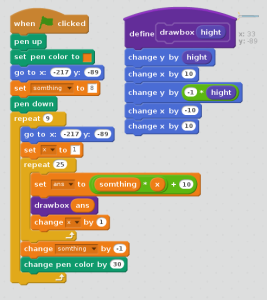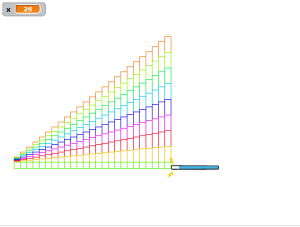## Learning Algebra With the the help of scratch

After finished Geometry i was switched to Algebra. Mastering algebra is important for moving on to nearly all other types of mathematics in  school. However, even the most basic algebra skills can be tricky for beginners to understand the first time they encounter them. I was in the need to know their prior knowledge in algebra in order to build on that (if that was conceptual) or correct them (if that was misconception). So, I gave some equations to solve, asked some stories (Multiplication & Division) and gave them small puzzles  to understand their understandings in algebra.

Except few, all posses some sort of difficulties. Some of them could not able to interpret the question, some of them having difficulties in calculation part, Some were guessed the answers. From that i inferred they need more explanation to understand the concepts. So, i took them to computer lab to practise algebra using scratch programming. First, i gave an expression of 5x+10 and asked them to draw bar graphs in scratch,                                     where x = 1,2,3,4,…….10(with guidance).

Then, i asked them to find out the value of x in the following equation 5x+10=70.

<iframe allowtransparency=”true” width=”485″ height=”402″ src=”http://scratch.mit.edu/projects/embed/53042546/?autostart=false”

What I was  inferred, they could learn(understand) the things comparatively quicker by programming than doing it manually (as procedural). Hence i decided to add one more concept on that just like fun by asking them to draw multi-stair case like structure by modifying the program   ( underguidance).